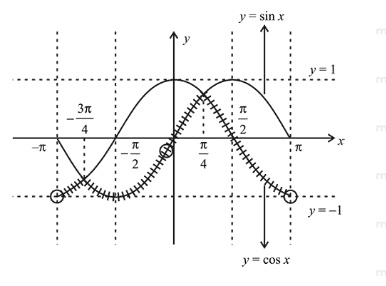# Let S be the set of all points inQuestion:

Let $S$ be the set of all points in $(-\pi, \pi)$ at which the function $f(x)=\min \{\sin x, \cos x\}$ is not differentiable. Then $S$ is a subset of which of the following?

1. (1) $\left\{-\frac{\pi}{4}, 0, \frac{\pi}{4}\right\}$

2. (2) $\left\{-\frac{3 \pi}{4},-\frac{\pi}{4}, \frac{3 \pi}{4}, \frac{\pi}{4}\right\}$

3. (3) $\left\{-\frac{\pi}{2},-\frac{\pi}{4}, \frac{\pi}{4}, \frac{\pi}{2}\right\}$

4. (4) $\left\{-\frac{3 \pi}{4},-\frac{\pi}{2}, \frac{\pi}{2}, \frac{3 \pi}{4}\right\}$

Correct Option: , 2

Solution:

$f(x)=\min \{\sin x, \cos x\}$$\because f(x)$ is not differentiable at $x=-\frac{3 \pi}{4}, \frac{\pi}{4}$

$\therefore \quad S=\left\{-\frac{3 \pi}{4}, \frac{\pi}{4}\right\}$

$\Rightarrow \quad S \subseteq\left\{-\frac{3 \pi}{4}, \frac{-\pi}{4}, \frac{3 \pi}{4}, \frac{\pi}{4}\right\}$